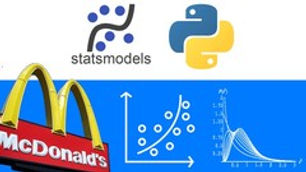# Introduction to Machine Learning with Case Study in Python#### Development & IT

19/7/20, 9:21 am

Understand concepts of least square regression and Hypothesis testing to our model like t-test, ANOVA, F-test, R square

Price:

\$0

##### EXPIRED

Description

In this course, you will learn concepts of linear regression. Starting from

• Case Study on Big Mac

• Statistical Questions

• Least Square Regression

• Hypothesis testing: t- test

• ANOVA and F-test

• Correlation

• R Square

You will learn the approaches towards regression with case study.  First we start with understanding linear equation and the optimization function value sum of squared errors.  With that we find the values of the coefficient and makes least square regression. Then we starts building our linear regression in python.

For the model we build we necessary test like hypothesis testing.

• t-test for coefficient significance

• ANOVA and F-test for model significance.

And finally, we answer the question statically. Hope we are seeing you inside the course !!!